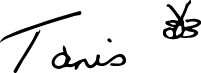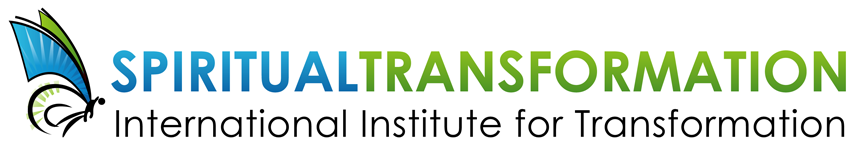# JANUARY 2020

 /* styles */ Dear Friend,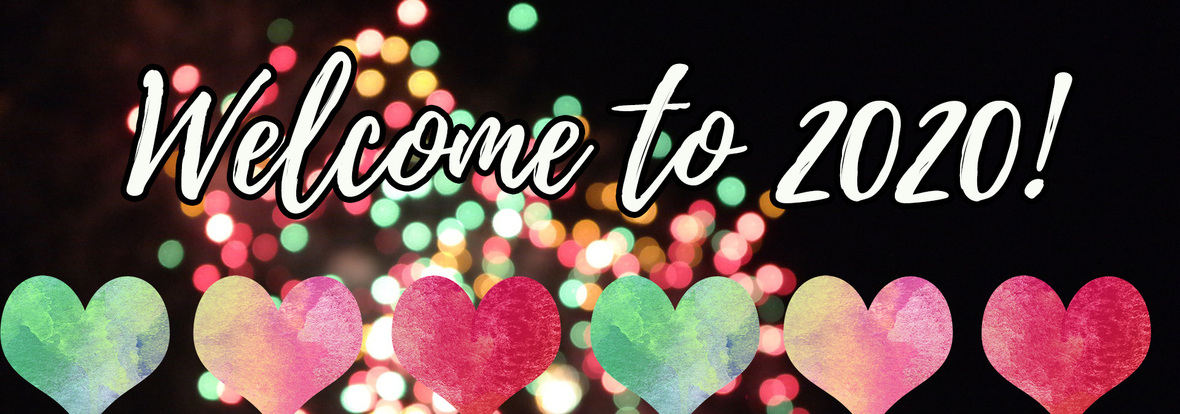/* styles */ I'd love to assist you in reaching your goals for 2020. If you weren't able to participate in my live YouTube Q&A session last month on goal setting for the new year, view the recording now and let's prepare for 2020 together. This chilly winter season is the perfect time to join me for a self-healing workshop in Kauai this January - details below. Or, you may wish to work on your own healing at home with the help of my books and e-courses which are available at a special price for the month of January. I'm also offering a workshop closer to home in Victoria, Canada in March on the importance of working with nature spirits to heal the Earth. Welcome to the new year, everyone!
 table div table+table+table+table+table+table div table{width:100%;padding:0}table div table+table+table+table+table+table div table img{width:96.23%;padding:0;float:none}table div table+table+table+table+table+table div table td{width:100%;padding:0 1.88% 18px}/* styles */table div table+table+table+table+table+table+table+table div table{width:100%;padding:0}table div table+table+table+table+table+table+table+table div table img{width:96.23%;padding:0;float:none}table div table+table+table+table+table+table+table+table div table td{width:100%;padding:0 1.88% 18px}/* styles */## NEW VIDEO: A fresh new beginning

 /* styles */ Key pointers for reaching your goals in 2020
 table.module-10{width:99.81%;padding:0}table div table+table+table+table+table+table+table+table+table+table+table div table{width:99.81%;float:none;margin-left:auto;margin-right:auto;padding:0}table div table+table+table+table+table+table+table+table+table+table+table div table a{border:0 none;text-decoration:none}table div table+table+table+table+table+table+table+table+table+table+table div table img{width:100%!important;border:0 none;text-decoration:none}table div table+table+table+table+table+table+table+table+table+table+table div table td{width:100%;padding:0}/* styles */
 /* styles */ If you weren't able to join Tanis for last month's live question and answer session (or even if you did!), view the recorded session on YouTube here as she discusses goal setting for the new year: https://youtu.be/uQga2P-oMOo "All my best wishes for NEW BEGINNINGS in 2020, and blessings always!" --Tanis
 table div table+table+table+table+table+table+table+table+table+table+table+table+table div table{width:100%;padding:0}table div table+table+table+table+table+table+table+table+table+table+table+table+table div table img{width:96.23%;padding:0;float:none}table div table+table+table+table+table+table+table+table+table+table+table+table+table div table td{width:100%;padding:0 1.88% 18px}/* styles */# Healing with the Physical, Emotional, Mental and Spiritual BodyIn this workshop you will develop a deeper relationship with your body elemental—your body consciousness—to diagnose causes of physical, emotional, mental weaknesses and co-create with it for optimal health.

Topics will include:

▪ Learn about the mental and emotional causes of illness
▪ Heal fears that create energy blocks in your cellular-etheric body to increase your frequency
▪ Discover genetic and ancestral patterns to heal your family lineage
▪ Exercises to dissolve negative thoughtforms that cause illness and pain
▪ Find out how the mind and heart program the body
▪ Learn heart meditations to create harmonious resonance and entrainment for all systems.
▪ Practice techniques with others to remove energy blocks
▪ How self-healing leads to healing of others and the Earth
 ▪ Learn about the mental and emotional causes of illness
 ▪ Heal fears that create energy blocks in your cellular-etheric body to increase your frequency
 ▪ Discover genetic and ancestral patterns to heal your family lineage
 ▪ Exercises to dissolve negative thoughtforms that cause illness and pain
 ▪ Find out how the mind and heart program the body
 ▪ Learn heart meditations to create harmonious resonance and entrainment for all systems.
 ▪ Practice techniques with others to remove energy blocks
 ▪ How self-healing leads to healing of others and the Earth

KAUAI DATES:

January 24, 2020 - Talk: Ancestors and Elementals of Kauai
Kapa’a , Kauai, Hawaii
Time: 7:30 to 9 pm
Fee: \$20
Register: Tracey Schavone, traceyschavone(at)gmail.com, 1-808-822-4465

January 25, 2020 - Workshop: Self-Healing with the Body Elemental
near Kapa’a, Kauai, Hawaii
Time: 9:30 am to 4:30 pm
Fee: \$125
Register: Tracey Schavone, traceyschavone(at)gmail.com, 1-808-822-4465

 table div table+table+table+table+table+table+table+table+table+table+table+table+table+table+table+table+table+table div table{width:100%;padding:0}table div table+table+table+table+table+table+table+table+table+table+table+table+table+table+table+table+table+table div table img{width:96.23%;padding:0;float:none}table div table+table+table+table+table+table+table+table+table+table+table+table+table+table+table+table+table+table div table td{width:100%;padding:0 1.88% 18px}/* styles */## BOOK SPECIALS - 20% off in January

Make this the year of your spiritual transformation journey with the help of these two wonderful books by Tanis Helliwell:

# The High Beings of Hawaii: Encounters with mystical ancestors

 table div table+table+table+table+table+table+table+table+table+table+table+table+table+table+table+table+table+table+table+table+table div table,table.module-20{width:43.02%;float:left;padding:0}table div table+table+table+table+table+table+table+table+table+table+table+table+table+table+table+table+table+table+table+table+table div table a{border:0 none;text-decoration:none}table div table+table+table+table+table+table+table+table+table+table+table+table+table+table+table+table+table+table+table+table+table div table img{width:100%!important;border:0 none;text-decoration:none}table div table+table+table+table+table+table+table+table+table+table+table+table+table+table+table+table+table+table+table+table+table div table td{width:100%;padding:0 20px 20px 0}/* styles */ Can’t go to Hawaii right now? Take a virtual trip with Tanis’ new book High Beings of Hawaii: Encounters with mystical ancestors. This new book for 2019 is perfect for winter reading during the frosty season! This Hawaiian vacation turns into a humorous, heart-opening odyssey that is alive with beings from other dimensions. Enjoy my newest book in either paperback or Kindle format.
 table div table+table+table+table+table+table+table+table+table+table+table+table+table+table+table+table+table+table+table+table+table+table+table div table{width:100%;padding:0}table div table+table+table+table+table+table+table+table+table+table+table+table+table+table+table+table+table+table+table+table+table+table+table div table img{width:96.23%;padding:0;float:none}table div table+table+table+table+table+table+table+table+table+table+table+table+table+table+table+table+table+table+table+table+table+table+table div table td{width:100%;padding:0 1.88% 18px}/* styles */# Manifest Your Soul's Purpose: The essential guide for life and work

 table div table+table+table+table+table+table+table+table+table+table+table+table+table+table+table+table+table+table+table+table+table+table+table+table+table+table+table div table{width:100%;padding:0}table div table+table+table+table+table+table+table+table+table+table+table+table+table+table+table+table+table+table+table+table+table+table+table+table+table+table+table div table img{width:96.23%;padding:0;float:none}table div table+table+table+table+table+table+table+table+table+table+table+table+table+table+table+table+table+table+table+table+table+table+table+table+table+table+table div table td{width:100%;padding:0 1.88% 18px}/* styles */# March 13, 14 & 15 | Victoria, BC Canada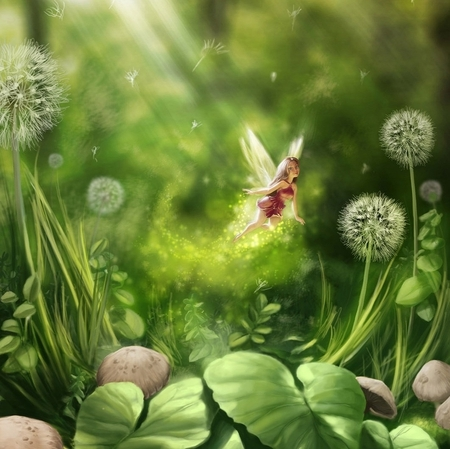/* styles */ March 13, 2020 - TALK & BOOK SIGNING: Why Working with Nature Spirits and Ancestors are Essential Today! Church of Truth, Victoria, http://cotvictoria.ca/ Time: 7:30-9:30pm Fee: \$20 at door Contact: Iris Scruton, wangchiaoyin(at)gmail.com Phone: 250-889-0042 March 14/15, 2020 - WORKSHOP: Humans to Work & Play with Nature Spirits to HEAL the EARTH Place: McTavish Academy of Art (https://mctavishacademy.ca/), 1720 McTavish Rd, North Saanich, BC Time: 9:30am to 5pm Saturday and 9:30 to 4 on Sunday Fee: \$245. Participants bring their own lunch for Saturday and bring a dish to share for Sunday potluck lunch. Tea, coffee, and snacks are provided during AM/PM breaks. Contact: Iris Scruton, wangchiaoyin(at)gmail.com Phone: 250-889-0042 To register for the workshop send payment by E-transfer to wangchiaoyin(at)gmail.com
 table div table+table+table+table+table+table+table+table+table+table+table+table+table+table+table+table+table+table+table+table+table+table+table+table+table+table+table+table+table+table+table+table div table{width:100%;padding:0}table div table+table+table+table+table+table+table+table+table+table+table+table+table+table+table+table+table+table+table+table+table+table+table+table+table+table+table+table+table+table+table+table div table img{width:96.23%;padding:0;float:none}table div table+table+table+table+table+table+table+table+table+table+table+table+table+table+table+table+table+table+table+table+table+table+table+table+table+table+table+table+table+table+table+table div table td{width:100%;padding:0 1.88% 18px}/* styles */## JANUARY E-COURSE SPECIALS

 /* styles */ We want to help you to get off to a good start in January and are offering these two e-courses to help you. Two of our most popular e-courses are available for US\$127 each for the month of January (regularly US\$147). Start a study group by contacting us at info(at)iitransform.com.

# Transform Yourself | Transform Your Work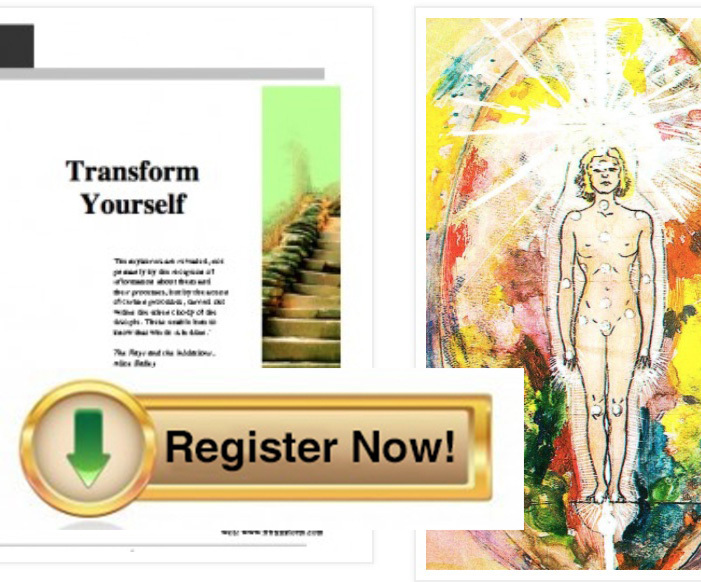TRANSFORM YOURSELF: To become conscious, we must develop spiritual intelligence and undergo a deep spiritual transformation. We need to let go of old patterns that no longer serve us, and unite our personality with our soul’s purpose. Learn how in this self-study e-course.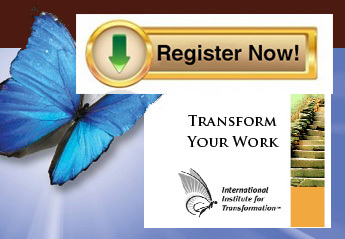TRANSFORM YOUR WORK: This ecourse helps you develop your spiritual intelligence (SQ). Bring your soul to work to achieve your life purpose, improve your relationships, have a happier work environment and have greater peace and joy.
 table div table+table+table+table+table+table+table+table+table+table+table+table+table+table+table+table+table+table+table+table+table+table+table+table+table+table+table+table+table+table+table+table+table+table+table+table+table+table div table{width:100%;padding:0}table div table+table+table+table+table+table+table+table+table+table+table+table+table+table+table+table+table+table+table+table+table+table+table+table+table+table+table+table+table+table+table+table+table+table+table+table+table+table div table img{width:96.23%;padding:0;float:none}table div table+table+table+table+table+table+table+table+table+table+table+table+table+table+table+table+table+table+table+table+table+table+table+table+table+table+table+table+table+table+table+table+table+table+table+table+table+table div table td{width:100%;padding:0 1.88% 18px}/* styles */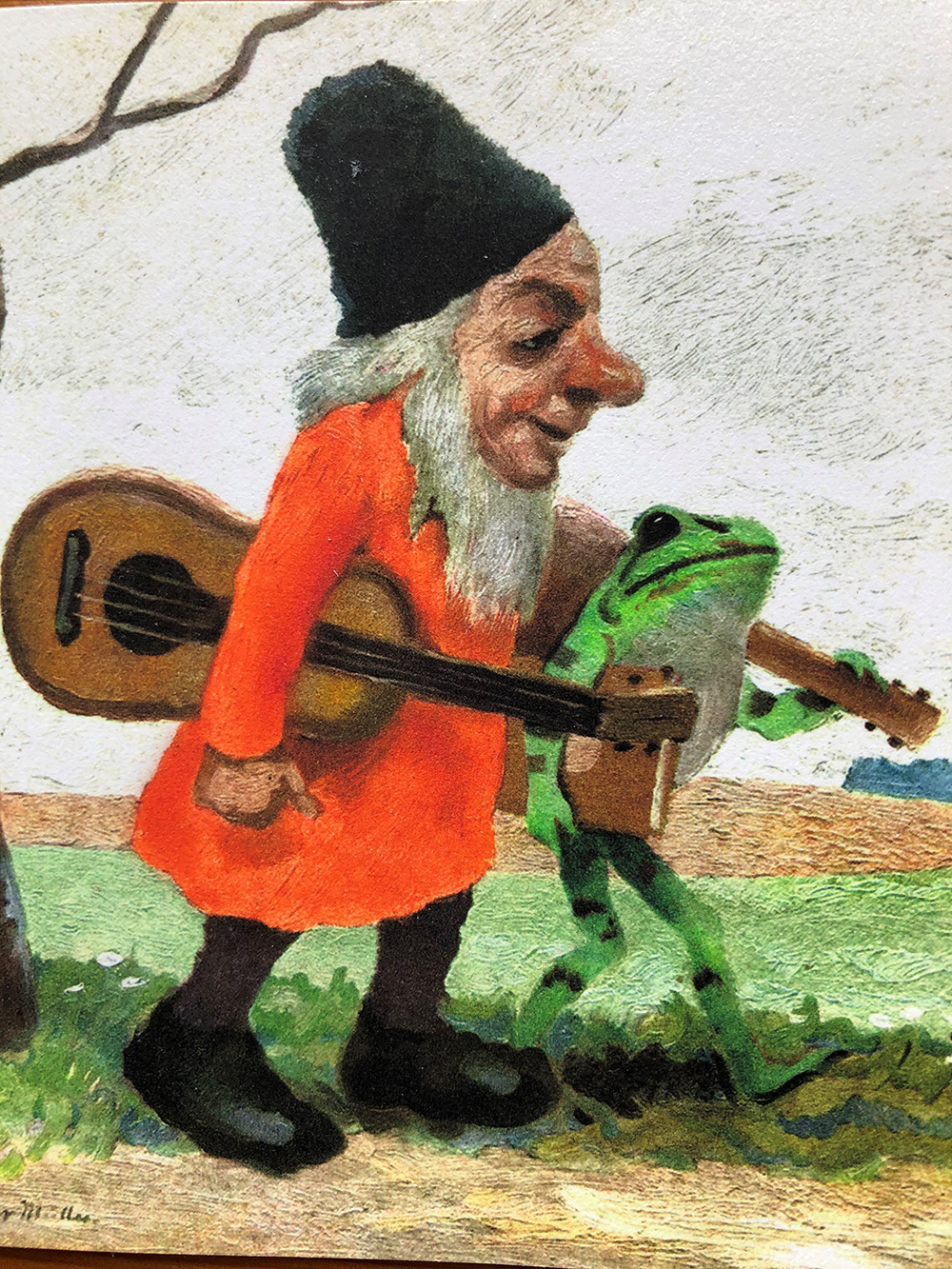table div table+table+table+table+table+table+table+table+table+table+table+table+table+table+table+table+table+table+table+table+table+table+table+table+table+table+table+table+table+table+table+table+table+table+table+table+table+table+table+table+table+table div table{width:100%;padding:0}table div table+table+table+table+table+table+table+table+table+table+table+table+table+table+table+table+table+table+table+table+table+table+table+table+table+table+table+table+table+table+table+table+table+table+table+table+table+table+table+table+table+table div table img{width:96.23%;padding:0;float:none}table div table+table+table+table+table+table+table+table+table+table+table+table+table+table+table+table+table+table+table+table+table+table+table+table+table+table+table+table+table+table+table+table+table+table+table+table+table+table+table+table+table+table div table td{width:100%;padding:0 1.88% 18px}/* styles */